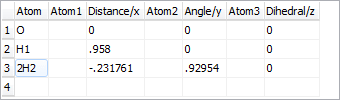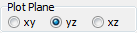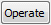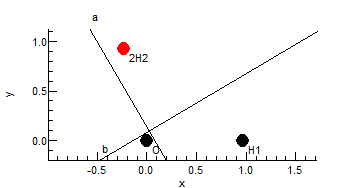Molecule Types Vibrational Structure Force Field Analysis

# z Matrix

The z matrix object is used to specify the the geometry of the molecule using the z matrix format commonly used by ab initio programs or standard Cartesian coordinates (or a mixture of the two). The units used are atomic mass units, Angstroms and degrees, independent of the units setting in the simulation object. The z matrix window, shown below for C2H4, allows the display and editing of this geometry. Provided the z matrix object is set active, changes in this window will be reflected in the Nuclear co-ordinates objects. To create a z matrix object, right click on the Electronic State object object and select "Add New..., z Matrix". To bring up the window right click on the z matrix object and select "View...".### The Controls

The grid at the top allows the z matrix to be edited; the example shown below shows a different molecule (HOD) using the alternate format where Cartesian coordinates are specified. Note that the grid will expand automatically as atoms are added.The "Plot Plane" control allows the selection of the plane in which to plot the molecule.The operate button brings up a menu of possible operations:
 Check Check the contents of the z matrix grid, and update the plot to match. Note that the Nucleus objects for the state are not updated immediately by this - Apply to State (below) will force this, or running a full calculation if the z matrix is active. Print Check the z matrix, and display information on the geometry, moments of inertia and principal axes in the Log Window. Apply to State Update the Nucleus objects for the state to reflect the current z matrix. This is only required if the Active property of the z matrix is false. Load from State Clear the z matrix, and set from the Nucleus objects for the state. This will use Cartesian format.The plot shows the geometry of the molecule, with the principal inertial axes displayed as for HOD above. The plot updates semi-automatically; use "Operate, Check" to force an update. The atom of currently selected row of the grid is highlighted in red.The status bar shows the latest mouse position; draw a box with the mouse to measure distance and angles.

## z Matrix Settings

 Active Set true to use this z matrix for geometry, in which case the Nuclear co-ordinates objects are updated from the z matrix. There must be no more than one active z matrix at a time. AverageMass If true, use the average mass of a nucleus if the isotope is not specified. If false, the exact mass of the most abundant isotope is used.

## z Matrix Format

The format required for the z matrix is as used by many ab initio programs, and elsewhere, and it should be possible to copy the z matrix to and from other programs with minimal or no editing.

### Atoms

The atoms (in the first column) can be specified in several formats:
• The standard is [Isotope]<Element Symbol>[Number] (such as 13C2), with the isotope and number optional. The number is arbitrary, and is used to distinguish different atoms of the same element. If the isotope is omitted, either the most abundant isotope, or the average mass is used, depending on the AverageMass setting. Dummy atoms (with zero mass) can be specified with Q or X for the element symbol. The masses are taken from http://physics.nist.gov/PhysRefData/Compositions/index.html.
• An integer is interpreted as an atomic number
• A number with a decimal point is interpreted as an exact mass.

The even numbered columns allow up to three connected atoms to be specified; the name used should appear in the first column of one of the rows above. If these are all blank, As a special case, on the first line where no linked atom can be specified, the second column (if not blank) is taken as specifying the plane to place the first three atoms in. The first letter specifies the axis on which the first two atoms lie, and the second character (if present) completes the specification of the plane.

### Specifying Bond Lengths and Angles

The odd numbered columns specify a distance (in Angstroms) and up to two angles (in degrees). The first angle (in column 5) is required after the second atom specifies the angle between the first three atoms on the line. For atoms after the third a dihedral angle must also be specified, which gives the angle between the planes containing the first three and last three atoms

The bond lengths and angles can be given as simple numerical values, but can also be given as general expressions involving symbolic values. This is typically more useful, as it allows symmetry constraints to be added naturally. For the C2H4 example above, note the use of two bond length variables (rCC and rCH) and a bond length variable (HCC). These can be used in expressions if required - an expression such as  180-HCC/2 might be needed in other contexts. When scanning the z matrix, unknown variables are automatically created as parameters to the current z matrix object, and they can then be edited in the Constants Window. If dealing with more than one isotopologue, these variables are better placed in a variables object, (with Global set to true) so the same value can be used in all species.

### Specifying Cartesian Coordinates

If all the linked atoms in a row are blank, then Cartesian format is assumed, and x, y and z should be given in the odd numbered columns. Cartesian coordinates can be mixed with the alternative format.

In Cartesian format, the rotation to the standard axis order in the principal axis system can be disabled by specifying 'norot' in the Atom1 position on the first line. This is ignored if the axes are not parallel to the principal axes.